# How to Combine Texts in Multiple Rows into One Cell Quickly in Excel

If we have data displays in multiple rows but one column, and we want to combine them into one single cell, normal copy and paste function doesn’t work. Except copy cells into one cell one by one, is there any other good idea? Actually, we can combine texts in multiple rows into one cell by formula, just use the combination of some functions, it can be implemented easily. This free tutorial will show you the formula and you just need to follow below steps to learn the way to combine rows.

Precondition:

See screenshot below. I entered some words in multiple rows. Now I want to move them into one cell.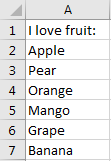## Method: Combine Multiple Rows into One Cell by Formula

Step 1: Select one cell to output the sentence combined with the words in rows. For example, select B1, then enter the formula =TRANSPOSE(A1:A7), then press F9. Verify that ={“I love fruit:”,”Apple”,”Pear”,”Orange”,”Mango”,”Grape”,”Banana”} is displayed in formula bar.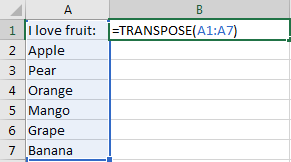After pressing F9: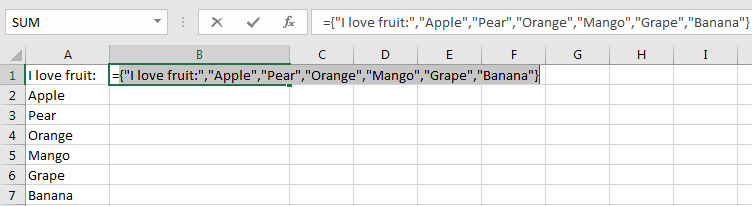Step 2: In the formula bar, update formula =CONCATENATE(“I love fruit:”,”Apple”,”Pear”,”Orange”,”Mango”,”Grape”,”Banana”). Based on previous formula, add CONCATENATE function, and remove curly brackets.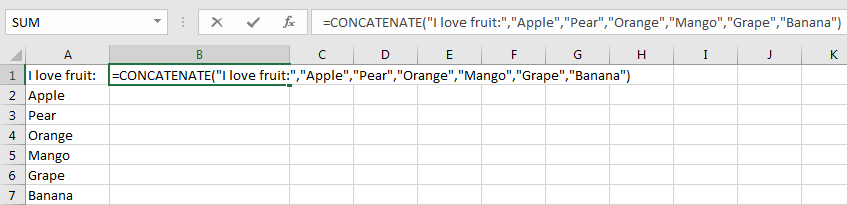Step 3: Press Enter to check result.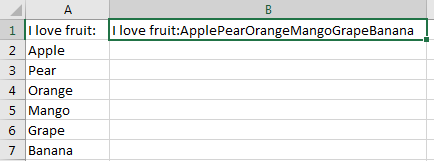Verify that words in different rows are combined into one cell properly, but obviously there is no separator between each word. If you want to separate them by space, you have to add “ “ between each word. You have to update formula =CONCATENATE(“I love fruit:”,” “,”Apple”,” “,”Pear”,” “,”Orange”,” “,”Mango”,” “,”Grape”,” “,”Banana”).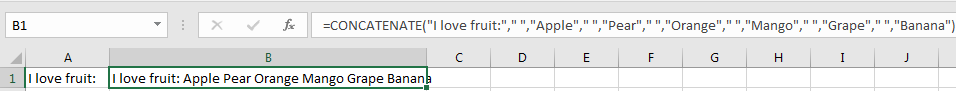Related Posts

If Cell is This Value or That Value

IF function is frequently used in Excel worksheet to return you expect “true value” or “false value” based on the result of logical test. If you want to see if a cell is A or B, and if one of ...

If Value is Greater Than A Certain Value

IF function is frequently used in Excel worksheet to return you expect “true value” or “false value” based on the logical test result. If you want to see if a value in one cell is greater than a specific value, ...

If Cell is Not Blank

IF function is frequently used in Excel worksheet to return you expect “true value” or “false value” based on the result of created logical test. If you want to see if a cell is blank or not, and leave some ...

VBA Macro For VLOOKUP From Another Sheet

In the previous post, you should know that how to fix or remove the #N/A error when using VLOOKUP formula to lookup value from another sheet. And this post will show you how to use VBA code to vlookup data ...

If Cell is Blank

IF function is frequently used in Excel worksheet to return you expect “true value” or “false value” based on the result of created logical test. If you want to see if a cell is blank or not, and leave some ...

If Cell Equals Certain Text String

IF function is frequently used in Excel worksheet to return you expect “true value” or “false value” based on the result of created logical test. If you want to see if cell equals a certain text string like “Win”, you ...

If Cell Contains Either Text1 or Text2

IF function is frequently used in Excel worksheet to return “true value” or “false value” based on the logical test result. If you want to see if cell contains certain substring1 like “abc” or substring2 like “def”, and returns true ...

If Cell Contains Certain Text OR Equals Certain Text

IF cell equals certain text IF function is frequently used in Excel worksheet to return “true value” or “false value” based on the logical test result. If you want to test values to see if they equal certain text like ...

VLOOKUP From Another Sheet Not Working

In the previous post, you should know that how to fix or remove the #N/A error when using VLOOKUP formula to lookup value from another sheet. And this post will show you reasons why your VLOOKUP formula is not working ...

If Cell Begins with One of Three Supplied Characters

If you want to test values to see if they begin with some given specific characters like “x”, ”y”, or “z”, you can create a formula with COUNTIF and SUM functions to return results. EXAMPLE You can see “TRUE” or ...

Sidebar